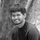cancel
Showing results for
Did you mean:Frequent Visitor

## total ytd of active employee in month end having start and end date

Have a table EmpActivity with Columns Employee Number,Start Date,End Date.Created a disconnected table CalenderAuto.

It is used as Slicer for Year.Need to get the Active employee YTD count on each month end of selected year.If the employee is currently working  End Date is Blanl

My output must be like

eg.2017 is selected

 Month Count Ytd Jan 10 10 Feb 8 18 Mar 10 28

1 ACCEPTED SOLUTIONCommunity Support

Hi, @Minu_Latha

Based on your description, you may create a calculated column and measures as below. The pbix file is attached in the end.

Calculated column:

``YearMonth = YEAR([Date])*100+MONTH([Date])``

Measure:

``EndOfMonth = MAX('Calendar'[Date])``
``````ECount =
var startdate = MIN('Calendar'[Date])
var enddate = MAX('Calendar'[Date])
var res =
CALCULATE(
DISTINCTCOUNT('BI EmpActivity'[BI.Personnel.EmpNo1]),
FILTER(
ALLSELECTED('BI EmpActivity'),
NOT(
OR(
[FromDate]>enddate,
[ToDate]<startdate
)
)
)
)
return
res``````
``````YTD =
var t = ALLSELECTED('BI EmpActivity')
var t1 =
SUMMARIZE(
ALLSELECTED('Calendar'),
'Calendar'[YearMonth],
"Result",
var startdate = MIN('Calendar'[Date])
var enddate = MAX('Calendar'[Date])
var res =
CALCULATE(
DISTINCTCOUNT('BI EmpActivity'[BI.Personnel.EmpNo1]),
FILTER(
t,
NOT(
OR(
[FromDate]>enddate,
[ToDate]<startdate
)
)
)
)
return
res
)
return
SUMX(
FILTER(
t1,
[YearMonth]<=MAX('Calendar'[YearMonth])&&
LEFT([YearMonth],4)=LEFT(MAX('Calendar'[YearMonth]),4)
),
[Result]
)``````

Result:Best Regards

Allan

If this post helps, then please consider Accept it as the solution to help the other members find it more quickly.

12 REPLIES 12Community Support

Hi, @Minu_Latha

Based on your description, you may create a calculated column and measures as below. The pbix file is attached in the end.

Calculated column:

``YearMonth = YEAR([Date])*100+MONTH([Date])``

Measure:

``EndOfMonth = MAX('Calendar'[Date])``
``````ECount =
var startdate = MIN('Calendar'[Date])
var enddate = MAX('Calendar'[Date])
var res =
CALCULATE(
DISTINCTCOUNT('BI EmpActivity'[BI.Personnel.EmpNo1]),
FILTER(
ALLSELECTED('BI EmpActivity'),
NOT(
OR(
[FromDate]>enddate,
[ToDate]<startdate
)
)
)
)
return
res``````
``````YTD =
var t = ALLSELECTED('BI EmpActivity')
var t1 =
SUMMARIZE(
ALLSELECTED('Calendar'),
'Calendar'[YearMonth],
"Result",
var startdate = MIN('Calendar'[Date])
var enddate = MAX('Calendar'[Date])
var res =
CALCULATE(
DISTINCTCOUNT('BI EmpActivity'[BI.Personnel.EmpNo1]),
FILTER(
t,
NOT(
OR(
[FromDate]>enddate,
[ToDate]<startdate
)
)
)
)
return
res
)
return
SUMX(
FILTER(
t1,
[YearMonth]<=MAX('Calendar'[YearMonth])&&
LEFT([YearMonth],4)=LEFT(MAX('Calendar'[YearMonth]),4)
),
[Result]
)``````

Result:Best Regards

Allan

If this post helps, then please consider Accept it as the solution to help the other members find it more quickly.Frequent Visitor

I need to know something to complete my work on YTD.
I have another table EmpInactivehistory with FromDate and this date have relation with calendar(date) Created.
I already have EmpActivity table which has no relationship with Calendar(date). Count & YTD is calculated from this Table based on count of employees at each month end (already done)
But the existing relationship causes the dates to be filtered out.
For the year 2019
EmpInactiveHistory have only 1 record in June
But year 2019
Empactivity based Count exists for all months.But Showing only 1 month(June) YTD.RelationShipWhen Relationship between Calendar(date &EmpInactiveHistory(FromDate) Removed output  from Empactivity is correctFormula for Employee Count from EmpActivity based on month endFrequent Visitor

It works fine Many ThanksSuper User

Connect Calendar Table with your main Fact table which contains all the data by creating a relationship. This will enable you to perform all the calculations involving dates.

Then to view a table with the months and the number of candidates, select the table visual and add Month from Calendar Table and a newly created measure [NoOfEmployees] which would look something like:

``NoofActiveEmployees = CALCULATE(COUNTROWS(TableName),filter(TableName,TableName[EndDate] <> BLANK())``

Try and see if it gives you the desired resultFrequent Visitor

My EmpActivity table have Start & End Date.So relationship cant be established

Emp No     Start Date        End Date       Activity

1                 05-02-2020    20-03-2020     Leave

2                 01-01-2020                            Still working

So when Slicer Year Selected 2020

Month     Empcount     YtdCount

Jan            1                    1

Feb            2                    3

Mar            1                   4

Need to get output like this .What to doSuper User

Can you provide your code for the Date Table?Frequent Visitor

More DetailsSuper User

Hello @Minu_LathaFrequent Visitor

Sorry Its not the desired output.Non blank records cannot be considered as active.The employee is active till now thats the meaning of End Date is nullSuper User

Yes, you are right.

Modify the measure as below:

``NoofActiveEmployees = CALCULATE(COUNTROWS('BI EmpActivity'),filter('BI EmpActivity','BI EmpActivity'[ToDate] = BLANK()))``

To verify this thing, you can create a calcultaed column using the code:

``Active/Inactive = If('BI EmpActivity'[ToDate] = Blank(), "Active","Inactive") ``Super User

Hi @Minu_Latha ,

Could you please explain it in more datailed manner? So that it would be helpful for me to understand and solve.

Thanks,

-Arul

Regards,
Arul
If I answered your question kindly mark my post as a solution and a kudo would be appreciated.Frequent Visitor

EmpActivity table contains Employee Details

Employee No

Start Date

End Date

Created Table Calendar using Calendarauto having no relation with EmpActivity

Year from Calendar used as slicer.Get the employee count in each month end & its ytd in each month